Start typing, then use the up and down arrows to select an option from the list.# Energy Diagrams Concept 3

Jules Bruno
271views
3
now looking at an energy diagram, we can also talk about stability of the chemical reaction itself. Here, we're going to say that stability is the difference in overall energy between the reactions and products and can determine the favorability. So how likely is this chemical reaction to occur for any type of chemical reaction? Now here, we're going to say that our overall energy, which is Delta E equals products minus react. It's now remember, for any typical energy diagram, our reactions are at the beginning, and our products are at the end. And we've kind of understood this idea of products minus reactant. For those of you have seen my videos on thermal chemistry. We've heard of this before when dealing with entropy, which is Delta H. This is when the overall energy is classified as thermal energy. So basically, instead of just writing the term energy where it's a broad idea of energy, where it could be thermal energy, cosmic energy, um, nuclear energy, you could be more specific and say, Delta H. In this case, we'd be saying that we're talking about specifically about thermal energy so you can have Delta e here or Delta H year. Same thing. Now here we said it Products minus reactions. If we take a look here, we can see that our product line here is at 10 killer jewels and then our reacted line looks like it's around 30 killer jewels. Subtracting them gives us negative 20 killer jewels. So here my difference in energy is negative. 20 killer jewels. A negative sign here for Delta E would also mean a negative sign for Delta H. So since we can interchange them, remember, a negative Delta H sign means that we're dealing with an exotic, thermic process. Remember, Exile Thermic means that our reaction releases energy. This is why our reactant are higher in energy and our products are lower in energy. Releasing the energy means that we're forming products that are lower in energy. On the other side, we have our product line. Looking like it's around 50, and then our reactant line looks like it's around. I'd say 15 or so. So here, when we subtract those two from each other, that gives us 35 positive. 35. Now we're going to say here that it's positive, which means it's endo thermic for Delta H. Remember Endo. Thermic processes mean we absorb energy. So are reacting to absorb energy, and as a result, we form products that are higher in energy. So that's why the curve moves up to represent the product line at the end. Okay, so keep this in mind. We can look at overall energy to give us an idea of what's more favorable. And in terms of these two graphs, it's always better to have products that are lower in energy. Because remember, in chemistry, lower energy means greater stability. So this chart this first chart, which has a negative delta p value or you can say as an exile thermic process, would be more stable overall than the other graph, which shows an increase in energy and an endo thermic process. Okay, so keep that in mind when looking at stability and energy diagrams.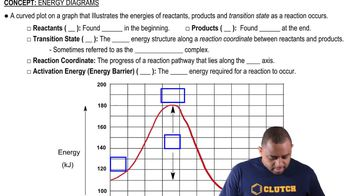01:57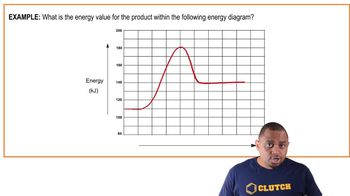01:02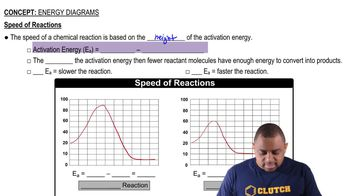02:02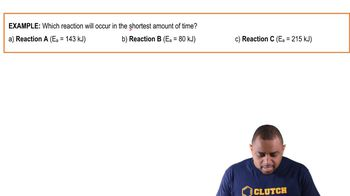00:40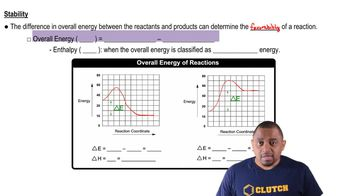03:22